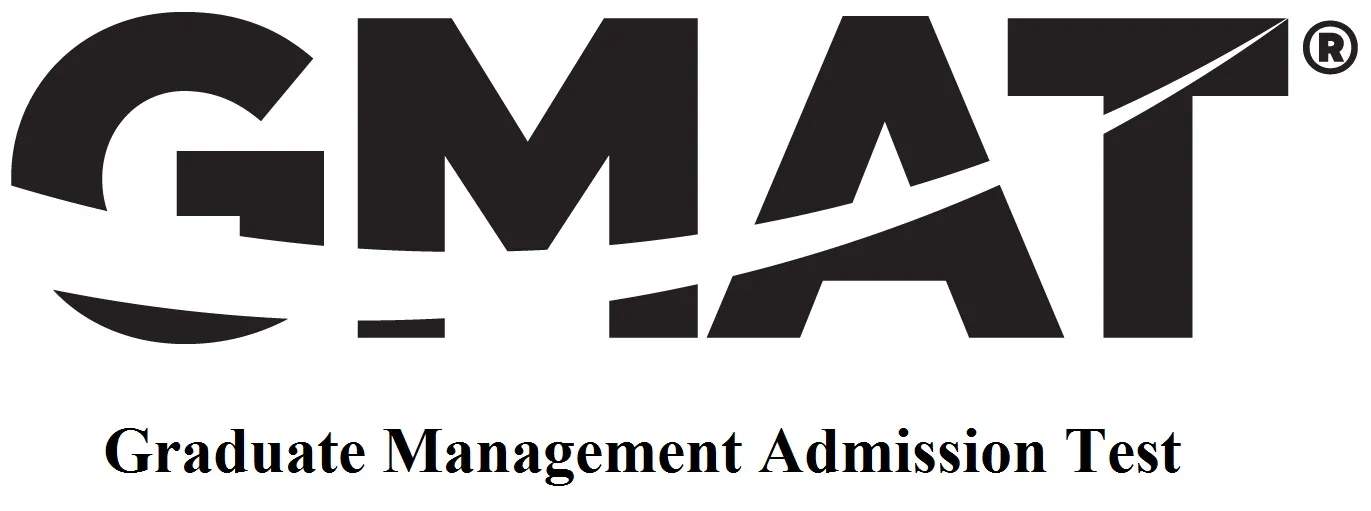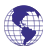## gmatprincipally in the United States, but also in other English-speaking countries. It is delivered via computer at various locations around the world. In those international locations where an extensive network of computers has not yet been established, the GMAT is offered either at temporary computer-based testing centers on a limited schedule or as a paper-based test (given once or twice a year) at local testing centers.
The exam measures verbal, mathematical, and analytical writing skills that the examinee has developed over a long period of time in his education and work. Test takers answer questions in each of the three tested areas, and there are also two optional breaks. In general, the test takes about four hours to complete. Scores are valid for five years (at most institutions) from the date the test taker sits for the exam until the date of matriculation (i.e. acceptance, not until the date of application).The maximum score that can be achieved on the exam is 800. The Analytical Writing Assessment (AWA) section is the first section to be answered. Then the Quantitative section and the Verbal Ability section follow respectively.

Analytical Writing Assessment

The Analytical Writing Assessment (AWA) section of the test consists of two essays. In the first, the student must analyze an argument and in the second the student must analyze an issue. Each essay must be written within 30 minutes and is scored on a scale of 0–6. The essay is read by two readers who each mark the essay with a grade from 0–6, in 0.5 point increments with a mean score of 4.1. If the two scores are within one point of each other, they are averaged. If there is more than one point difference, the essays are read by a third reader. The first reader is IntelliMetric, a proprietary computer program developed by Vantage Learning, which analyzes creative writing and syntax of more than 50 linguistic and structural features. The second and third readers are humans, who evaluate the quality of the examinee's ideas and his ability to organize, develop, and express ideas with relevant support. While mastery of the conventions of written English factor into scoring, minor errors are expected, and evaluators are trained to be sensitive to examinees whose first language is not English. Each of the two essays in the Analytical Writing part of the test is graded on a scale of 0 (the minimum) to 6 (the maximum):

•An essay that is totally illegible or obviously not written on the assigned topic.
•An essay that is fundamentally deficient. 2: An essay that is seriously flawed.
•An essay that is seriously limited.
•An essay that is merely adequate
•An essay that is strong.
•An essay that is outstanding.

Quantitative Section

The quantitative section consists of 37 multiple choice questions, which must be answered within 75 minutes. There are two types of questions: problem solving and data sufficiency. The quantitative section is scored from 0 to 51 points.

Problem Solving

This tests the quantitative reasoning ability of the examinee. Problem-solving questions present multiple-choice problems in arithmetic, basic algebra, and elementary geometry. The task is to solve the problems and choose the correct answer from among five answer choices. Some problems will be plain mathematical calculations; the rest will be presented as real life word problems that will require mathematical solutions.Numbers: All numbers used are real numbers.
Figures: The diagrams and figures that accompany these questions are for the purpose of providing useful information in answering the questions. Unless it is stated that a specific figure is not drawn to scale, the diagrams and figures are drawn as accurately as possible. All figures are in a plane unless otherwise indicated.

Data Sufficiency

This tests the quantitative reasoning ability using an unusual set of directions. The examinee is given a question with two associated statements that provide information that might be useful in answering the question. The examinee must then determine whether either statement alone is sufficient to answer the question; whether both are needed to answer the question; or whether there is not enough information given to answer the question. Data sufficiency is a unique type of math question created especially for the GMAT. Each item consists of the questions itself followed by two numbered statements.

•If statement 1 alone is sufficient to answer the question, but statement 2 alone is not sufficient.
•If statement 2 alone is sufficient to answer the question, but statement 1 alone is not sufficient.
•If both statements together are needed to answer the question, but neither statement alone is sufficient.
•If either statement by itself is sufficient to answer the question.
•If not enough facts are given to answer the question.

Perhaps the easiest way to fully internalize the scope of these questions is to replace the word “is” with the words “must be” - the questions are not asking whether an answer is possible, but rather, whether it "must" be the case.

Verbal Section

The verbal section consists of 41 multiple choice questions, which must be answered within 75 minutes. There are three types of questions: sentence correction, critical reasoning, and reading comprehension. The verbal section is scored from 0 to 51 points.

Sentence Correction

The Sentence Correction section tests a test taker's knowledge of American English grammar, usage, and style. Sentence correction items consist of a sentence, all or part of which has been underlined, with five associated answer choices listed below the sentence. The first answer choice is exactly the same as the underlined portion of the sentence. The remaining four answer choices contain different phrasings of the underlined portion of the sentence. The test taker is instructed to choose the first answer choice if there is no flaw with that phrasing of the sentence. If there is a flaw with the original phrasing of the sentence, the test taker is instructed to choose the best of the four remaining answer choices. Sentence Correction questions are designed to measure a test taker's proficiency in three areas: correct expression, effective expression, and proper diction. Correct expression refers to the grammar and structure of the sentence. Effective Expression refers to the clarity and concision used to express the idea. Proper Diction refers to the suitability and accuracy of the chosen words in reference to the dictionary meaning of the words and the context in which the words are presented.

Critical Reasoning

This tests logical thinking. Critical thinking items present an argument that the test taker is asked to analyze. Questions may ask test takers to draw a conclusion, to identify assumptions, or to recognize strengths or weaknesses in the argument. It presents brief statements or arguments and asks to evaluate the form or content of the statement or argument. Questions of this type ask the examinee to analyze and evaluate the reasoning in short paragraphs or passages. For some questions, all of the answer choices may conceivably be answers to the question asked. The examinee should select the best answer to the question, that is, an answer that does not require making assumptions that violate common sense standards by being implausible, redundant, irrelevant, and inconsistent.